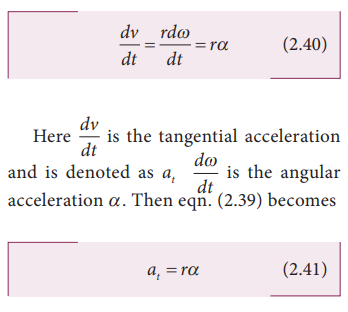Home | | Physics 11th std | Angular displacement in Projectile Motion

# Angular displacement in Projectile Motion

Consider a particle revolving around a point O in a circle of radius r.

Angular displacement

Consider a particle revolving around a point O in a circle of radius r (Figure 2.45). Let the position of the particle at time t = 0 be A and after time t, its position is B.Then,

The angle described by the particle about the axis of rotation (or center O) in a given time is called angular displacement.

i.e., angular displacement = AOB =θ

The unit of angular displacement is radian.

The angular displacement (θ) in radian is related to arc length S (AB) and radius r as## Angular velocity(Vector ω−>)

The rate of change of angular displacement is called angular velocity.

If θ is the angular displacement in time t, then the angular velocity ω isThe unit of angular velocity is radian per second (rad s−1). The direction of angular velocity is along the axis of rotation following the right hand rule. This is shown in Figure 2.46.## Angular acceleration (α)

The rate of change of angular velocity is called angular acceleration.The angular acceleration is also a vector quantity which need not be in the same direction as angular velocity.

## Tangential acceleration

Consider an object moving along a circle of radius r. In a time t, the object travels an arc distance s as shown in Figure 2.47. The corresponding angle subtended is Δθwhich gives the relation between linear speed and angular speed.

Equation (2.38) is true only for circular motion. In general the relation between linear and angular velocity is given byFor circular motion equation (2.39) reduces to equation (2.38) sinceandare perpendicular to each other.

Differentiating the equation (2.38) with respect to time, we get (since r is constant)The tangential acceleration at experienced by an object is circular motion as shown in Figure 2.48.Note that the tangential acceleration is in the direction of linear velocity.

Study Material, Lecturing Notes, Assignment, Reference, Wiki description explanation, brief detail
11th Physics : UNIT 2 : Kinematics : Angular displacement in Projectile Motion |